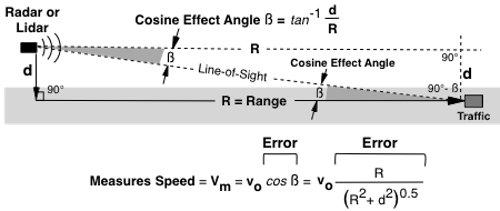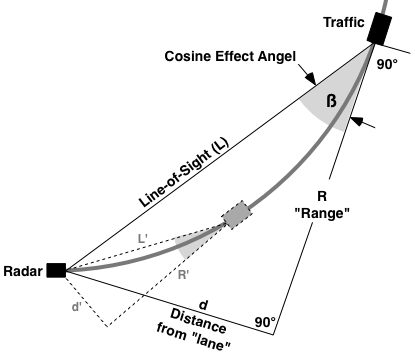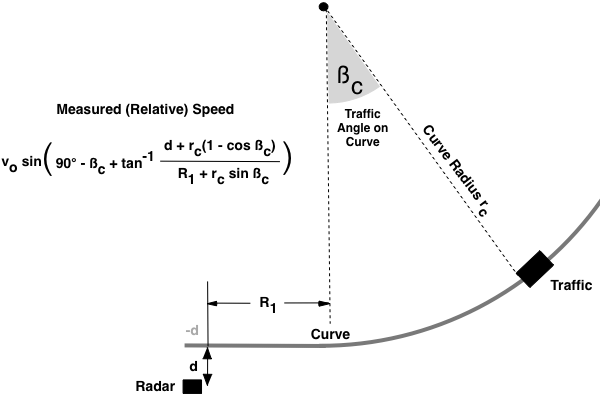Previous | Next

# Cosine Effect Error on Radar and Lidar

Contents -- Setup | Curves | Graph
 Cosine Effect Setup

Police microwave and laser radars measure the relative speed a vehicle is approaching, or receding, the radar. If a vehicle is traveling directly at the radar the relative speed is actual speed. If the vehicle is not traveling directly at the radar the relative speed is slightly lower than actual speed. The phenomenon is called the Cosine Effect because the measured speed is directly related to the cosine of the angle between the radar and vehicle direction of travel or speed vector. The greater the angle the greater the speed error and the lower the measured speed. A cosine angle of 90° has 100% error, speed measures zero.

Cosine Effect Setupvo = Actual Speed a = Cosine Effect Angle R = Target Range to Radar d = Antenna Distance to Middle of Target Lane

CALCULATORs
 Measured (Cosine)Speed from Angle Actual Speed: MPH KPH Knots ft/s units Cosine Angle: Degrees Measured Speed
 Measured (Cosine) Speed from Setup Actual Speed: MPH Target Range: feet Radar Distance to Lane: feet Measured Speed Speed Drop Rate Cosine Effect Angle

The cosine effect angle is the angle between the radar antenna and the vehicle direction of travel. The angle depends on vehicle range and radar antenna distance from vehicle lane center. The antenna pointing angle is completely irrelevant, only the angle to the target vehicle matters.

The cosine function is always between 0 and 1. The cosine of 0° is 1, measured speed is actual speed. The cosine of 90° is 0, measured speed is zero. The closer the angle is to 0° the more accurate the speed measured.

The cosine effect applies to both microwave and laser radars. Photo radars are Across the Road Radars that angle the beam across the road, typically 20° to 23°. As a vehicle passes through the beam the measured speed is changing and spreading due to the cosine effect. The changing and spreading of the measured speed in a very short time makes photo radars inherently less accurate than Down the Road microwave and laser radars.

 Overpass

The cosine effect has 2 components if the radar or lidar is elevated to traffic such as from an overpass. Radar distance from traffic lane is a function of horizontal and vertical distances.

Cosine Effect from an OverpassIn the figure, "x" is the horizontal distance to the vehicle lane center, and "y" is the vertical distance to the average top of the target vehicle. Radar distance to vehicle path is "d" and equals the square root of the sum of "x" squared plus "y" squared. If either the horizontal or vertical component is zero, the equations reduce to that shown above.

 Traffic on a Curve

The cosine effect angle on curves is large and changing proportional to curve radius and vehicle speed. The relatively large and fast changing cosine effect angle results in measured speed changing relatively fast, too fast in most scenarios for a radar or lidar to measure speed.

Changing Cosine Effect AngleMicrowave and laser radars cannot measure traffic speed on a curve because the angle is changing causing the relative speed to change too fast for the radar or lidar to measure.

Measured Speed on a CurveMeasured Speed Variables

• Vehicle Speed (vo)
• Vehicle Angle on Curve (ßc)
• Curve Radius (rc)
• Randar Range to curve start/end (R1)
• Radar Distance to Vehicle Lane (d)

 Cosine Error Graph

The below figure is a graphical representation of the Cosine Effect for measured speed as a percentage of true speed versus angle. The larger the angle the larger the error and the lower the relative target speed. At angles of only a few degrees the speed is 99% to 100% of actual, at an angle of 60° the speed is half actual speed. At 90° speed is 0 relative to the radar or lidar.

Cosine Effect Angle vs Measured Speed %